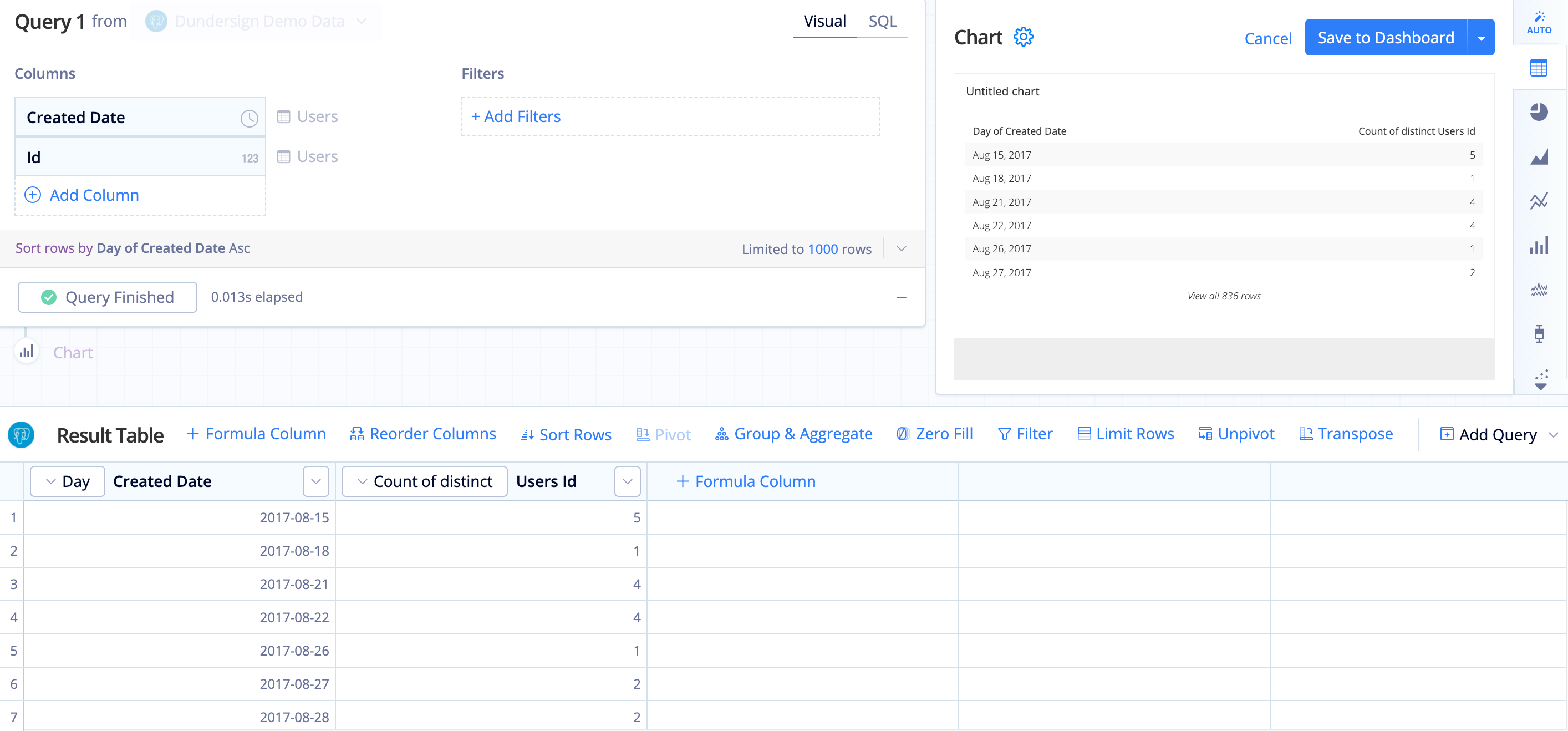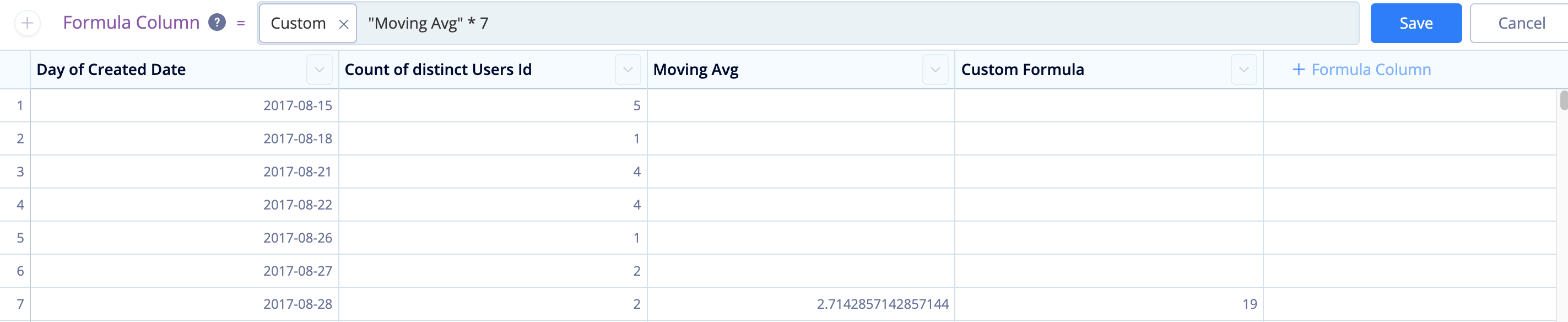# Calculate a rolling sum in Visual SQL

You can calculate a moving average in Chartio by using our Moving Average guided formula in the Pipeline using a Formula Column or Apply Formula Action. In the event you’d like to calculate a rolling sum (or moving sum), you can easily edit the Moving Average to multiply it by the selected period.

In the example below, we create a chart that pulls the count of users per day. Here, we also want to include a column that calculates the rolling sum of the count of users in the last seven days. This is different from a standard running total because it only includes the sum of the previous seven rows of data.

To do this, follow the steps below:

1. Start by building a chart for the count of users per day.

Here, we use our Dundersign Demo Data data source and add the Created Date and Id columns from the Users table to our Query, keeping the grouping and aggregation as Day and Count of distinct, respectively. Click Run Query.2. Add a new column to your result set to calculate the moving average.

Click Formula Column above the Result Table, select moving average from the list of guided formulas. For the parameters, choose Count of distinct Users Id as the input column and type `7` for the number of trailing rows to calculate the moving average for the last seven days. Click Save.

Note: If you don’t have rows for every day and want to make sure you calculate null values for the last week, add a Zero Fill Action before the Formula Column Action.

3. The moving average is simply the sum of the last seven values divided by 7. Therefore, we can get our rolling sum by multiplying the moving average by seven.

Add another Formula Column to do exactly that. Simply select Custom as the formula type and use the following formula, replacing `moving_average` with your custom column from Step 2.

`````` "moving_average" * 7
``````4. For clarity, rename this column to `Rolling Sum`.
5. Since it’s no longer necessary, hide the Moving Avg column from your result set.
6. Switch your chart to a Line chart from the chart types to visualize your daily user count compared to the rolling user sum. Don’t forget to save your chart to your dashboard when done.